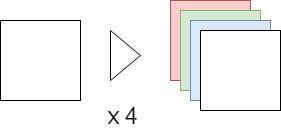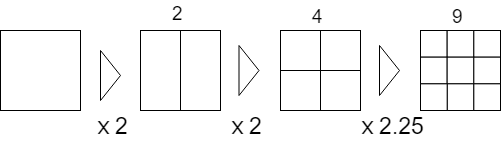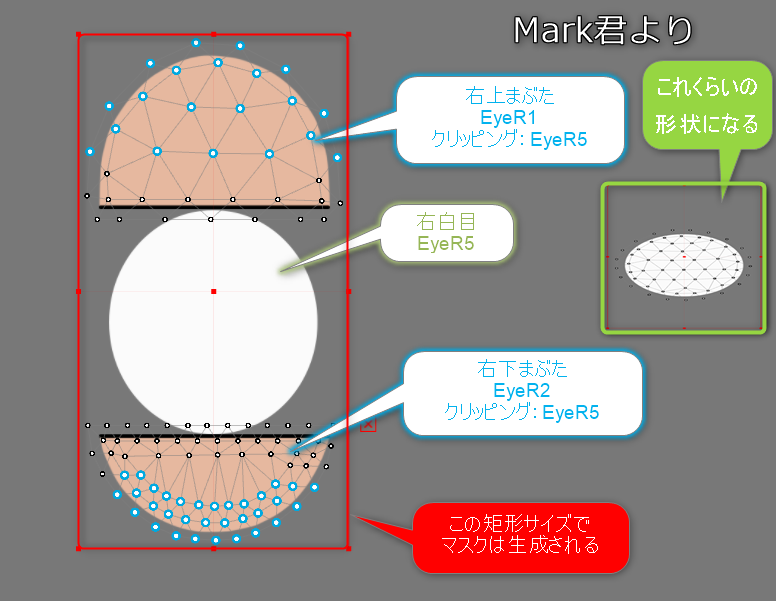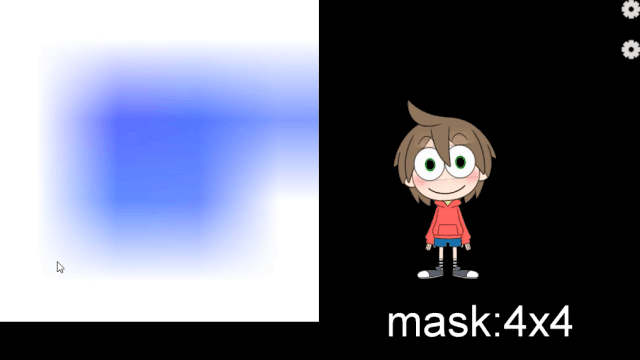Updated: 02/21/2023

In order to maintain drawing speed on smartphones and other devices, the Live2D Cubism SDK for Native uses the “preprocessing method” in which all mask shapes are drawn in a single mask buffer at the beginning of the model drawing process.

In the general drawing method, the mask shape is drawn each time a Drawable that requires a mask is drawn (see figure).
This method would result in a relatively expensive process of switching render targets, clearing buffers, etc. each time the Drawable needs a mask.
This may cause slow rendering speeds on smartphones and other devices.

To solve this problem, the following processing can be performed on a single mask buffer to minimize memory usage while treating it as if multiple mask buffers were used.

Since all masks are generated in advance, Drawables with the same mask specification can use the same mask image to reduce the number of masks to be generated.

This is done, in the CubismRenderer_OpenGLES2::Initialize function call, by the CubismClippingManager_OpenGLES2::Initialize function.

```void CubismClippingManager_OpenGLES2::Initialize(CubismModel& model, csmInt32 drawableCount, const csmInt32** drawableMasks, const csmInt32* drawableMaskCounts)
{
// Register all drawable objects that use clipping masks
// Clipping masks should normally be used only for a few pieces
for (csmInt32 i = 0; i < drawableCount; i++)
{
{
// ArtMeshes without clipping masks (often not used)
_clippingContextListForDraw.PushBack(NULL);
continue;
}

// Check if it is the same as an already existing ClipContext
if (cc == NULL)
{
// Generate if the same mask does not exist.
}

_clippingContextListForDraw.PushBack(cc);
}
}```

## Use of Multiple Mask Textures

In Cubism SDK for Native R6 beta1 or later, you can optionally use multiple textures for masks.
Therefore, even if a model is set with more masks than the maximum number of masks (36) that existed up to R5, the model will be displayed correctly in the SDK.

However, if two or more mask textures are used, the maximum number of masks generated for each mask texture is 32.
(The maximum number of masks is 36 when only one mask is used. Details are described below.)
If two mask textures are used, the maximum number of masks that can be used is 32 × 2, or 64.

The settings for using multiple textures for masks are as follows.

```int maskBufferCount = 2;

CubismRenderer renderer = Rendering::CubismRenderer::Create();

If nothing is passed to the second argument of CubismRenderer::Initialize(), only one texture for the mask will be generated and used.

`renderer->Initialize(cubismModel);`

## Separation by Color Information

The mask buffer is an RGBA video array, just like a normal texture buffer, etc.
The normal mask process uses only this A channel to apply the mask, but not the RGB channels.
Therefore, by having separate mask data for R, G, B, and A, one mask buffer can be treated as four mask buffers.## Separation by Dividing

When 4 mask images are not enough, the number of masks can be increased by handling the mask buffer in 2, 4, or 9 divisions.
Combined with the separation by color information, up to 36 (4 x 9) different masks can be held.Also, to prevent the mask image from being crushed, the mask is drawn on all Drawable rectangles to which the mask is applied.
This requires range generation as well as matrix generation for the mask generation and the use of masks.When multiple mask textures are used, the division method is the same as when only one mask texture is used.
However, if multiple mask textures are used, the quality of the drawing of the same model will be improved because the allocation of masks to each mask texture will be equally divided as much as possible. (More textures for masks will cost more.)
For example, if a model has 32 masks, normally one mask texture is used to try to draw 32 masks, but if two mask textures are used, the mask allocation is “16 masks per texture.”

### Checking rectangles

In the first step of mask generation, for each mask, check the rectangle that fits all the mask application destinations.

```void CubismClippingManager_OpenGLES2::CalcClippedDrawTotalBounds(CubismModel& model, CubismClippingContext* clippingContext)
{
// The entire rectangle of the clipped mask (drawable object to be masked)
csmFloat32 clippedDrawTotalMinX = FLT_MAX, clippedDrawTotalMinY = FLT_MAX;
csmFloat32 clippedDrawTotalMaxX = FLT_MIN, clippedDrawTotalMaxY = FLT_MIN;

// Determine if this mask is actually needed
// If even one “Drawable Object” that uses this clipping is available, a mask must be generated

const csmInt32 clippedDrawCount = clippingContext->_clippedDrawableIndexList->GetSize();
for (csmInt32 clippedDrawableIndex = 0; clippedDrawableIndex < clippedDrawCount; clippedDrawableIndex++)
{
// Find the rectangle to be drawn for the drawable object that uses the mask
const csmInt32 drawableIndex = (*clippingContext->_clippedDrawableIndexList)[clippedDrawableIndex];

const csmInt32 drawableVertexCount = model.GetDrawableVertexCount(drawableIndex);
csmFloat32* drawableVertexes = const_cast<csmFloat32*>(model.GetDrawableVertices(drawableIndex));

csmFloat32 minX = FLT_MAX, minY = FLT_MAX;
csmFloat32 maxX = FLT_MIN, maxY = FLT_MIN;

csmInt32 loop = drawableVertexCount * Constant::VertexStep;
for (csmInt32 pi = Constant::VertexOffset; pi < loop; pi += Constant::VertexStep)
{
csmFloat32 x = drawableVertexes[pi];
csmFloat32 y = drawableVertexes[pi + 1];
if (x < minX) minX = x;
if (x > maxX) maxX = x;
if (y < minY) minY = y;
if (y > maxY) maxY = y;
}

//
if (minX == FLT_MAX) continue; // If a single valid point was not obtained, skip it.

// Reflected in the entire rectangle
if (minX < clippedDrawTotalMinX) clippedDrawTotalMinX = minX;
if (minY < clippedDrawTotalMinY) clippedDrawTotalMinY = minY;
if (maxX > clippedDrawTotalMaxX) clippedDrawTotalMaxX = maxX;
if (maxY > clippedDrawTotalMaxY) clippedDrawTotalMaxY = maxY;
}
if (clippedDrawTotalMinX == FLT_MAX)
{
clippingContext->_allClippedDrawRect->X = 0.0f;
clippingContext->_allClippedDrawRect->Y = 0.0f;
clippingContext->_allClippedDrawRect->Width = 0.0f;
clippingContext->_allClippedDrawRect->Height = 0.0f;
clippingContext->_isUsing = false;
}
else
{
clippingContext->_isUsing = true;
csmFloat32 w = clippedDrawTotalMaxX - clippedDrawTotalMinX;
csmFloat32 h = clippedDrawTotalMaxY - clippedDrawTotalMinY;
clippingContext->_allClippedDrawRect->X = clippedDrawTotalMinX;
clippingContext->_allClippedDrawRect->Y = clippedDrawTotalMinY;
clippingContext->_allClippedDrawRect->Width = w;
clippingContext->_allClippedDrawRect->Height = h;
}
}```

### Layout settings with color separation and divisional separation

Defines the color channel and divisional position of the mask buffer that each mask belongs to.

```void CubismClippingManager_OpenGLES2::SetupLayoutBounds(csmInt32 usingClipCount) const
{
// Layout masks using a single RenderTexture that is as full as possible
// If the number of mask groups is 4 or less, 1 mask is placed on each RGBA channel; if the number is between 5 and 6, 2 masks are in each RG channel and 1 mask is in each BA channel

// Use RGBA in order.
const csmInt32 div = usingClipCount / ColorChannelCount; // Basic number of masks to be placed on one channel.
const csmInt32 mod = usingClipCount % ColorChannelCount; // Allocate the remainder, one by one, to this numbered channel.

// Provide a channel for each RGBA (0: R, 1: G, 2: B, 3: A)
csmInt32 curClipIndex = 0; // Set in order.

for (csmInt32 channelNo = 0; channelNo < ColorChannelCount; channelNo++)
{
// Number of layouts for this channel
const csmInt32 layoutCount = div + (channelNo < mod ? 1 : 0);

// Determine the separation method
if (layoutCount == 0)
{
// Do nothing
}
else if (layoutCount == 1)
{
// Use everything as is
cc->_layoutChannelNo = channelNo;
cc->_layoutBounds->X = 0.0f;
cc->_layoutBounds->Y = 0.0f;
cc->_layoutBounds->Width = 1.0f;
cc->_layoutBounds->Height = 1.0f;
}
else if (layoutCount == 2)
{
for (csmInt32 i = 0; i < layoutCount; i++)
{
csmInt32 xpos = i % 2;

cc->_layoutChannelNo = channelNo;

cc->_layoutBounds->X = xpos * 0.5f;
cc->_layoutBounds->Y = 0.0f;
cc->_layoutBounds->Width = 0.5f;
cc->_layoutBounds->Height = 1.0f;
// Divide UV into 2 and use
}
}
else if (layoutCount <= 4)
{
// Divide into 4 and use
for (csmInt32 i = 0; i < layoutCount; i++)
{
csmInt32 xpos = i % 2;
csmInt32 ypos = i / 2;

cc->_layoutChannelNo = channelNo;

cc->_layoutBounds->X = xpos * 0.5f;
cc->_layoutBounds->Y = ypos * 0.5f;
cc->_layoutBounds->Width = 0.5f;
cc->_layoutBounds->Height = 0.5f;
}
}
else if (layoutCount <= 9)
{
// Divide into 9 and use
for (csmInt32 i = 0; i < layoutCount; i++)
{
csmInt32 xpos = i % 3;
csmInt32 ypos = i / 3;

cc->_layoutChannelNo = channelNo;

cc->_layoutBounds->X = xpos / 3.0f;
cc->_layoutBounds->Y = ypos / 3.0f;
cc->_layoutBounds->Width = 1.0f / 3.0f;
cc->_layoutBounds->Height = 1.0f / 3.0f;
}
}
else
{
CubismLogError("not supported mask count : %d", layoutCount);
}
}
}```

### Matrix generation for drawing and using masks

Prepare transformation matrices for mask generation and mask use based on the area and the location of the rectangle examined before drawing.

```           // --- Actually draw one mask. ---
csmRectF* allClippedDrawRect = clipContext->_allClippedDrawRect; // Enclose the logical coordinates of all drawable objects that use this mask in a rectangle.
csmRectF* layoutBoundsOnTex01 = clipContext->_layoutBounds; // Put the mask in this range.

// Use a rectangle on the model coordinates with appropriate margins.
csmFloat32 MARGIN = 0.05f;
_tmpBoundsOnModel.SetRect(allClippedDrawRect);
_tmpBoundsOnModel.Expand(allClippedDrawRect->Width * MARGIN, allClippedDrawRect->Height * MARGIN);
//########## Essentially, do not use the entire allocated area, but keep the size to the minimum necessary

// Calculate the formula for shaders. The following is the case when rotation is not considered
// movePeriod' = movePeriod * scaleX + offX		  [[ movePeriod' = (movePeriod - tmpBoundsOnModel.movePeriod)*scale + layoutBoundsOnTex01.movePeriod ]]
csmFloat32 scaleX = layoutBoundsOnTex01->Width / _tmpBoundsOnModel.Width;
csmFloat32 scaleY = layoutBoundsOnTex01->Height / _tmpBoundsOnModel.Height;

// Obtain the matrix to be used when generating the mask.
{
// Obtain the matrix to be passed to the shader <<<<<<<<<<<<<<<<<<<<<<<< Optimization required (can be simplified by calculating in reverse order)
{
// Translate Layout 0..1 to -1..1
_tmpMatrix.TranslateRelative(-1.0f, -1.0f);
_tmpMatrix.ScaleRelative(2.0f, 2.0f);
}
{
// view to Layout0..1
_tmpMatrix.TranslateRelative(layoutBoundsOnTex01->X, layoutBoundsOnTex01->Y); //new = [translate]
_tmpMatrix.ScaleRelative(scaleX, scaleY); //new = [translate][scale]
_tmpMatrix.TranslateRelative(-_tmpBoundsOnModel.X, -_tmpBoundsOnModel.Y);
//new = [translate][scale][translate]
}
// tmpMatrixForMask is the result of calculation
}

//--------- Calculate the matrix for mask reference at draw time
{
// Obtain the matrix to be passed to the shader <<<<<<<<<<<<<<<<<<<<<<<< Optimization required (can be simplified by calculating in reverse order)
{
_tmpMatrix.TranslateRelative(layoutBoundsOnTex01->X, layoutBoundsOnTex01->Y); //new = [translate]
_tmpMatrix.ScaleRelative(scaleX, scaleY); //new = [translate][scale]
_tmpMatrix.TranslateRelative(-_tmpBoundsOnModel.X, -_tmpBoundsOnModel.Y);
//new = [translate][scale][translate]
}

_tmpMatrixForDraw.SetMatrix(_tmpMatrix.GetArray());
}

clipContext->_matrixForDraw.SetMatrix(_tmpMatrixForDraw.GetArray());```

## Dynamic Resizing of Mask BuffersThe GLES2 renderer provides an API to resize the mask buffer at runtime.
Currently, the mask buffer size is initially set to 256*256 (pixels), but if the mask generation area is to be cut into 9 pieces, the mask shape drawn in an 85*85 (pixels) rectangle area will be further enlarged and used as the clipping area.

As a result, the edges of the clipping result are blurred or blotchy.
As a solution to this problem, an API is provided to change the size of the mask buffer at program execution time.

For example, if the mask buffer size is 256*256 => 1024*1024 and the mask generation area is cut into 9 pieces, the mask shape can be drawn in a rectangle area of 341*341, so when enlarged and used as a clipping area, it eliminates edge blurring or blotches.

Note: Increase the size of the mask buffer => The more pixels to be processed, the slower the speed, but the cleaner the drawing result.
Note: Reduce the size of the mask buffer => The fewer pixels to be processed, the faster the speed, but the drawing result will be less clean.

```void CubismRenderer_OpenGLES2::SetClippingMaskBufferSize(csmInt32 size)
{
// Destroy and recreate instances to resize the FrameBuffer
CSM_DELETE_SELF(CubismClippingManager_OpenGLES2, _clippingManager);

_clippingManager = CSM_NEW CubismClippingManager_OpenGLES2();

_clippingManager->Initialize(
*GetModel(),
GetModel()->GetDrawableCount(),
);
}```

## Why Preprocessing Methods Can Improve Performance

Specifically for mobile devices, the processing cost of the Clear instruction and rendering target switching instruction to the GPU may be higher than other instructions.
When drawing using the general method, these instructions with high processing costs are executed as many times as the number of Drawables that require masks.
However, with the preprocessing method, the number of times these instructions are executed can be reduced, resulting in improved performance in smartphones and other devices.

To understand the actual effect, let’s measure the time cost of each processing unit while rendering.
As a measurement method, check with the source code shown below. Layers are measured separately for each build.
The model being tested is a single Haru.
Two Android devices and one Windows device were prepared for the measurement.
The measurement policy is to use the clock_gettime function on the Android side and the QueryPerformanceCounter function on the Windows side to cache the results in a buffer and calculate the average value.

### Clipping mask generator (layer 1)

```void CubismClippingManager_OpenGLES2::SetupClippingContext(CubismModel& model, CubismRenderer_OpenGLES2* renderer)
{
・
・
・
{	// ★Measurement of drawing target switching.
P_TIME1(ProcessingTime cl(s_switch);)
// ---------- Mask drawing process -----------
// Set RenderTexture for masks to active
}

{	// ★Measurement of buffer clearing (fill process).
P_TIME1(ProcessingTime cl(s_clear);)
// (Temporary specification) 1 is invalid area (not drawn), 0 is valid area (drawn). (Make a mask by applying a value close to 0 with Cd*Cs in the shader. Nothing happens when multiplying by 1.)
glClearColor(1.0f, 1.0f, 1.0f, 1.0f);
glClear(GL_COLOR_BUFFER_BIT);
}
・
・
・
{	// ★Measurement of drawing target switching 2.
P_TIME1(ProcessingTime cl(s_switch);)
// --- Postprocessing ---
glBindFramebuffer(GL_FRAMEBUFFER, oldFBO); // Return the drawing target.
}
・
・
・
}```

CubismClippingManager_OpenGLES2::SetupClippingContext measures the time to switch and fill drawing targets.

### Entire model drawing (mixed layer 1 and 2)

```void CubismRenderer_OpenGLES2::DoDrawModel()
{

{	// ★Measurement of overall mask buffer generation.
if (_clippingManager ! = NULL)
{
PreDraw();
_clippingManager->SetupClippingContext(*GetModel(), this);
}
}

{　// ★Measurement of drawing preprocessing.
// Note that PreDraw is called once even within the clipping process above!!
PreDraw();
}

const csmInt32 drawableCount = GetModel()->GetDrawableCount();
const csmInt32* renderOrder = GetModel()->GetDrawableRenderOrders();

{　// ★Measure sort time.
// Sort index by draw order.
for (csmInt32 i = 0; i < drawableCount; ++i)
{
const csmInt32 order = renderOrder[i];
_sortedDrawableIndexList[order] = i;
}
}

{　// ★Measurement of drawing time other than masks.
// Draw.
for (csmInt32 i = 0; i < drawableCount; ++i)
{
const csmInt32 drawableIndex = _sortedDrawableIndexList[i];

SetClippingContextBufferForDraw((_clippingManager ! = NULL)
? (*_clippingManager->GetClippingContextListForDraw())[drawableIndex]
: NULL);

IsCulling(GetModel()->GetDrawableCulling(drawableIndex) ! = 0);

DrawMesh(
GetModel()->GetDrawableTextureIndices(drawableIndex),
GetModel()->GetDrawableVertexIndexCount(drawableIndex),
GetModel()->GetDrawableVertexCount(drawableIndex),
const_cast<csmUint16*>(GetModel()->GetDrawableVertexIndices(drawableIndex)),
const_cast<csmFloat32*>(GetModel()->GetDrawableVertices(drawableIndex)),
reinterpret_cast<csmFloat32*>(const_cast<Core::csmVector2*>(GetModel()->GetDrawableVertexUvs(drawableIndex))),
GetModel()->GetDrawableOpacity(drawableIndex),
GetModel()->GetDrawableBlendMode(drawableIndex)
);
}
}

{　// ★Measurement of drawing postprocessing.
//
PostDraw();
}
}```

Measure pre-draw, sort, and post-draw processing.

It is also measured in the broad framework of mask generation and other drawing by separating layers.

### Drawing mesh (layer 1)

```void CubismRenderer_OpenGLES2::DrawMesh(csmInt32 textureNo, csmInt32 indexCount, csmInt32 vertexCount
, csmUint16* indexArray, csmFloat32* vertexArray, csmFloat32* uvArray
, csmFloat32 opacity, CubismBlendMode colorBlendMode)
{
・
・
・
{　// ★Measure the data set time to the shader.
P_TIME1(ProcessingTime sharder(s_sharder);)
this, drawTextureId, vertexCount, vertexArray, uvArray
, opacity, colorBlendMode, modelColorRGBA, IsPremultipliedAlpha()
, GetMvpMatrix()
);
}

{　// ★Time measurement of a single drawing instruction.
P_TIME1(ProcessingTime gldraw(s_gldraw);)
// Draw a polygon mesh
glDrawElements(GL_TRIANGLES, indexCount, GL_UNSIGNED_SHORT, indexArray);
}

// Postprocessing
glUseProgram(0);
SetClippingContextBufferForDraw(NULL);
}```

CubismRenderer_OpenGLES2::DrawMesh measures the setup time to the shader and the single drawing instruction time.

### Layer 3

```void LAppModel::Update()
{
{
P_TIME3(ProcessingTime up(s_paramup);)
const csmFloat32 deltaTimeSeconds = LAppPal::GetDeltaTime();
_userTimeSeconds += deltaTimeSeconds;
・
・
・
// Pose settings
if (_pose ! = NULL)
{
_pose->UpdateParameters(_model, deltaTimeSeconds);
}
}
{
P_TIME3(ProcessingTime ren(s_modelup);)

_model->Update();

}
}```
```void LAppModel::Draw(CubismMatrix44& matrix)
{
P_TIME3(ProcessingTime ren(s_rendering);)

matrix.MultiplyByMatrix(_modelMatrix);

GetRenderer<Rendering::CubismRenderer_OpenGLES2>()->SetMvpMatrix(&matrix);

DoDraw();
}```

The Update flow is roughly divided into the following three categories: Parameter calculation, model update, and rendering.

### Result

 Android1 Android2 Winpc1 L1clear 1781.20 218.80 26.80 L1gldraw 45.47 51.63 10.58 L1sharder 12.31 9.34 5.37 L1post 1.50 1.00 0.10 L1switch 10.70 56.30 7.80 L1predraw 15.90 8.20 2.20 L1sort 7.60 7.00 0.60 L2MaskMake 2686.80 1357.60 318.50 L2draw 4004.10 4013.20 1217.00 L3paramupdate 392.00 375.40 89.70 L3modelupdate 1357.50 1410.90 1070.40 L3rendering 6715.70 5233.70 1892.00

The table above shows the execution times for the devices mentioned earlier.
You’ll find that the cost of Clear is high for mobile devices and that switching rendering targets is heavier than for other instructions.
When this heavy instruction draws in the general way, it runs as many Drawables as the mask will require.
This calculation only needs to be done once, which can be expected to improve performance on smartphones and other devices.

## Switching to a high-definition method for processing masks

As mentioned above, the method of generating a mask each time a drawing is made will affect performance on low-end devices.

However, this method is more suitable when screen quality is more important than performance at runtime, as in the case of final output as video.

In the SDK after 12/20/2018, the mask process can be switched to a high-definition method.
To switch to the high-definition method, pass true to the following API.

`CubismRenderer::UseHighPrecisionMask()`